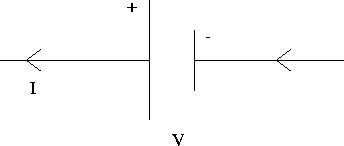# Circuits

We will now introduce some methods for analyzing electrical circuits, enabling us, for example, to predict the current through a given circuit element. The circuits we will be considering at this point include two types of elements:
• A source of the power in the circuit. For the DC (direct current) circuits we will be working with, this is a battery, such as that found in flashlights or cars. The rating of the battery in volts is called the electromotive force (emf) of the battery, and is sometimes given the symbolE . In a circuit it is represented as in Fig. 17.2.The convention used is that for a battery supplying power the current comes out of the positive terminal and reenters the negative one. However, when a battery is being charged the current flows in the opposite direction.

• a resistor, which is represented as Fig. 17.3.This can be an actual resistor placed in a circuit, or might be the resistance associated with another element in the circuit, such as the internal resistance of a battery.

Before discussing general methods for analyzing circuits, we first consider two special cases for which some simplifications occur.Next: Resistors in Series Up: Current and Resistance (Ch. Previous: Energy and Power